# 6. 附录：超级快速入门步骤

## 6.1. 搭建开发环境

```https://pan.baidu.com/s/1uzZ-Ic4XPclwRXgk5f-Z_g  提取码：3005
```

### 6.1.2. 安装开发板的驱动程序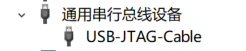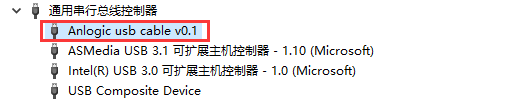### 6.1.3. 安装 Arduino IDE 并配置对 YADAN Board 的支持

```https://gitee.com/verimake/yadan_arduino/raw/master/package_verimake_core_index.json
```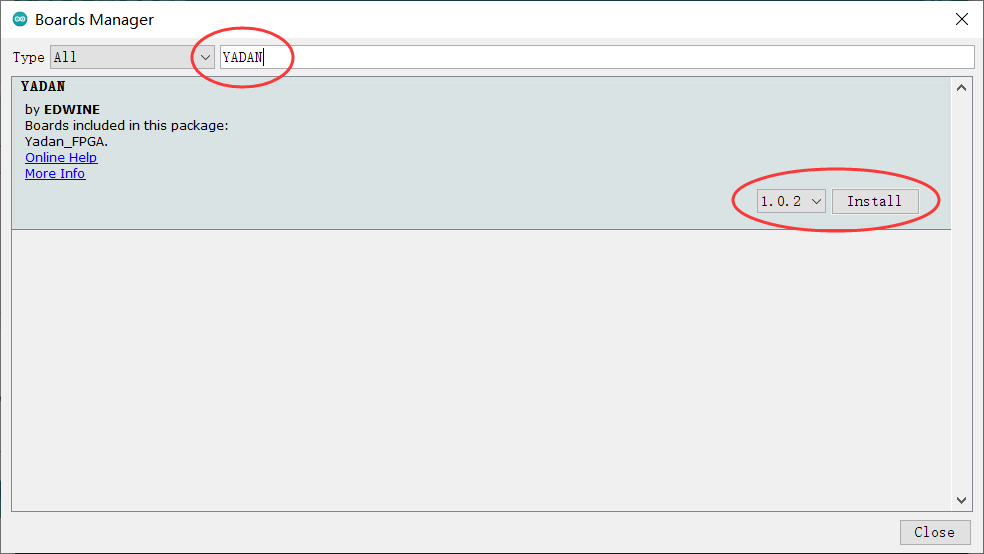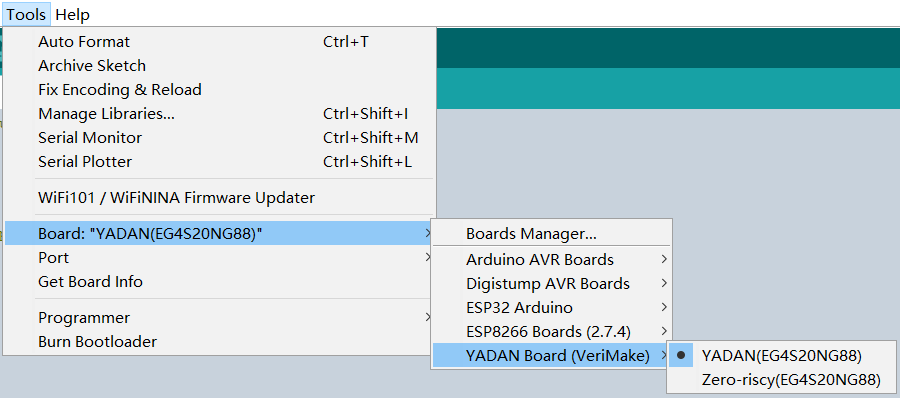### 6.1.4. 安装 RISC-V GCC 工具链

The xPack GNU RISC-V Embedded GCC 是一种 Windows 下可用的 RISC-V GCC 工具链，我们以这个为例来介绍安装和使用步骤。

```riscv-none-embed-gcc --version
```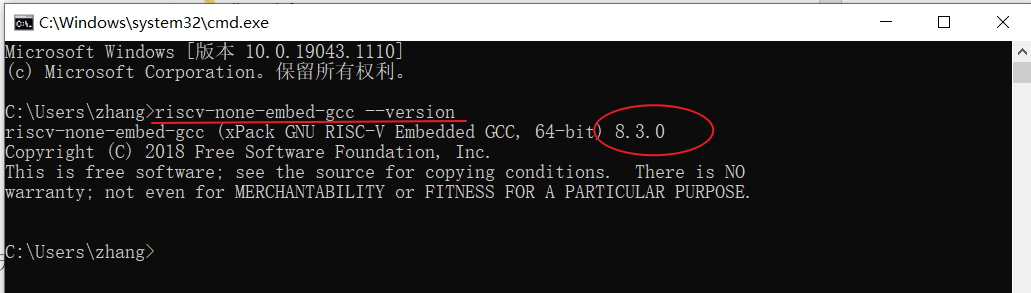### 6.2.1. 部署 SoC 至 FPGA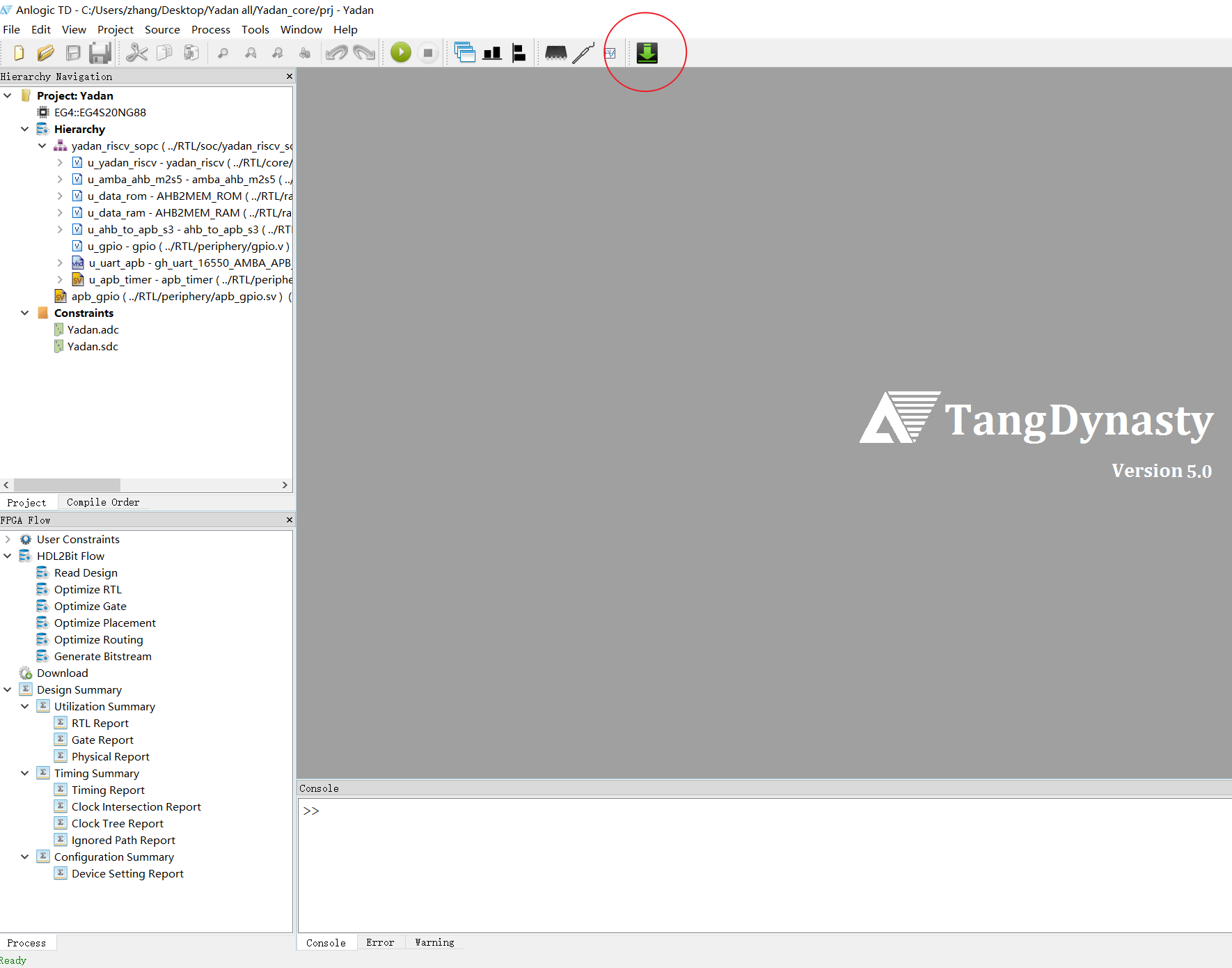### 6.2.2. 使用 Arduino IDE 开发应用程序

```/*
Turns an LED on for one second, then off for one second, repeatedly.
This example code is in the public domain: http://www.arduino.cc/en/Tutorial/Blink
*/

// the setup function runs once when you press reset or power the board
void setup()
{
// initialize digital pin LED_BUILTIN as an output.
pinMode(LED_BUILTIN, OUTPUT);
}

// the loop function runs over and over again forever
void loop()
{
digitalWrite(LED_BUILTIN, HIGH);   // turn the LED on (HIGH is the voltage level)
delay(1000);                       // wait for a second
digitalWrite(LED_BUILTIN, LOW);    // turn the LED off by making the voltage LOW
delay(1000);                       // wait for a second
}
```

### 6.2.3. 使用 RISC-V GCC 工具链从底层开发程序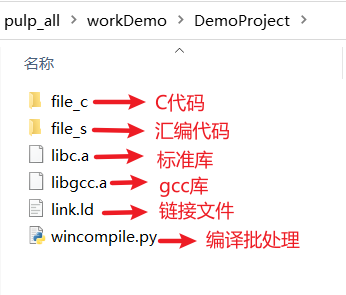`file_c``file_s` 分别用于存放 C 代码和汇编代码，启动文件已位于 `file_s` 中，是目前 SoC 适用的一个完整版，后期我们编写的自己的 C 代码只需放入 `file_c` 目录即可。`libc.a ``libgcc.a` 是库文件，`link.ld` 是链接脚本，它将程序首地址定位在 0x0000_0000，RAM 定位在 0x0010_0000。

```#define REG(add) (*(volatile unsigned int *)(add))  // 宏定义能让后边的代码更简短

int main()
{
PADDIR = 1 << 13; // 设置 GPIO 的第 13 号引脚为输出模式

while (1)         // 无限循环
{
PADOUT = 1 << 13;                // 设置 GPIO 的第 13 号输出高电平
for (int i = 0; i < 300000; i++) // 软件延时
;
PADOUT = 0 << 13;                // 设置 GPIO 的第 13 号输出低电平
for (int i = 0; i < 300000; i++) // 软件延时
;
}

return 1;
}
```

```python wincompile.py
```

1. 如果安装有 Python 环境，可使用 Python 直接运行 `.py` 源文件

```python YADANdownload.py comX 115200 simple.bin A
```
2. 如果使用 exe 文件，则为

```YADANdownload.exe comX 115200 simple.bin A
```